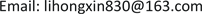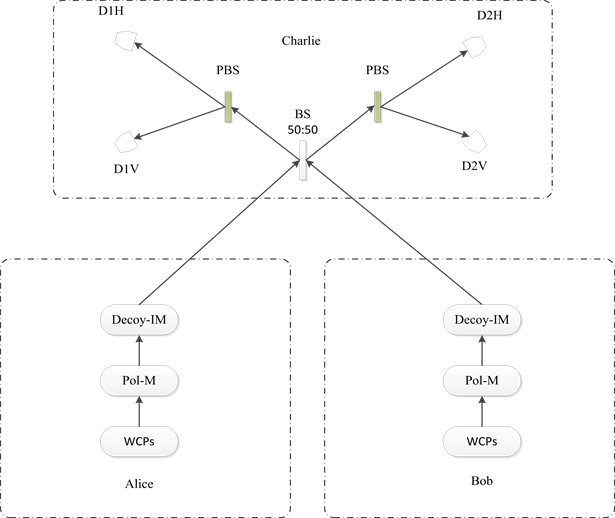﻿ 测量设备无关量子密钥分发方案安全性研究 Security Analysis on Measurement-Device-Independent Quantum Key Distribution Protocol

Modern Physics
Vol.07 No.06(2017), Article ID:22813,12 pages
10.12677/MP.2017.76030

Security Analysis on Measurement-Device-Independent Quantum Key Distribution Protocol

Hongxin Li1,2, Xiangbin Wang1, Xin Liu1, Yu Han1, Bao Yan1, Wei Wang1

1Luoyang Campus, Strategic Support Force Information Engineering University, Luoyang Henan

2State Key Laboratory of Mathematical Engineering and Advanced Computing, Zhengzhou HenanReceived: Nov. 6th, 2017; accepted: Nov. 20th, 2017; published: Nov. 27th, 2017ABSTRACT

Quantum key distribution (QKD) has been paid much attention because of its theoretical unconditional security. However, in the actual system, QKD will be vulnerable to Quantum hackers because of non-perfection of equipment. The proposal of the measurement device independent (MDI) QKD provide a good solution to this problem. In this paper, we firstly introduce the latest research progress and implementation principles of the MDI-QKD protocol. And then, we summarize the advantages and disadvantages of the protocol in the practical application. Based on the analysis of the MDI-QKD improvement protocol, our research is mainly focused on the method of lifting scheme secure key generation rate and theoretical proof.

Keywords:Quantum Key Distribution, Measurement Device Independent, Side-Channel Attack, HSPS

1战略支援部队信息工程大学洛阳校区，河南 洛阳

2数学工程与先进计算国家重点实验室，河南 郑州1. 引言

QKD是量子通信技术中实用化程度较高的应用之一，因其理论上的无条件安全性一直备受关注。1984年IBM公司的Charles H.Bennett和蒙特利尔大学的Gilles Brassard Bennett提出第一个QKD协议即BB84协议，由于简单高效而被广泛使用。然而，在实际使用过程中，由于设备的不完美性，量子密钥系统还存在着较多的漏洞。被称为量子黑客的攻击者可以针对这些漏洞，实施诸如分束攻击、时移攻击、致盲攻击等来获取密钥信息。而在这中间，探测器遭到了最为频繁的攻击。

2015年加拿大卡尔加里大学的量子科学技术研究所的Raju Valivarthi等人提出了更好的反馈机制，用以消除信道传输上的不足  。2015年，南京邮电大学毛钱萍等提出了基于波分复用技术的MDI-QKD协议，在不增加系统传输设备的前提下，提高了系统的密钥生成率  。2016年，中国科学技术大学的尹华磊等人使用最优化的四强度诱骗态方法进行了长距离MDI-QDK实验，利用超低损耗光纤的安全传输距离达到404 km，创造了新的安全传输记录  。2016年，空军工程大学的薛阳等人提出了一种基于修正相干态光源的MDI-QKD方案，相较于使用HSPS光源的系统，传输距离提高了9%  。2017年，空军工程大学的朱卓丹等人提出了一种基于预报相干光子对的MDI-QKD改进方案，降低了长距离量子密钥分发中由暗计数引起的误码率  。

2. MDI-QKD协议概述

2012年，Lo小组提出了MDI-QKD方案在抵御探测器的侧信道攻击方面取得了较好的成果。在MDI- QKD协议中，Alice和Bob准备任意BB84偏振态的随机弱相干脉冲(weak coherent pulses，简称WCPs)，并且将它们发送给一个处在中间位置的不可信的第三方Charlie，Charlie为了将接收到的信号变成Bell态会执行一个Bell态测量。Alice和Bob可以应用诱骗态技术来估计增益和量子比特错误率(quantum bit error rate，简称QBER)。

2.1. MDI-QKD协议流程

1) Alice和Bob独立地制备弱相干光源(WCPs)。Alice和Bob通过利用偏振调制器(Pol-M)来制备不同的BB84状态的随机WCPs并通过使用强度调制器(Decoy-IM)来生成诱骗态。完成以上操作后，Alice和Bob将会通过信道独立地将自己的信号发送给一个处于中间位置的不可信第三方Charlie。

2) 在测量过程中，Alice和Bob发送的信号脉冲进入到一个50:50的分束器(BS)中进行干涉，之后分别进入两个偏振分束器(PBS)中将输入光子投射成水平的(H)或垂直的(V)偏振态。

3) Charlie将执行一个Bell态测量用来将接收到的信号变成Bell态，该端的四个光子探测器用来探测结果。Bell状态测量是否成功与两个探测器的测量结果有关。当测量设备不可信时，通过此过程Alice

4) Alice和Bob所需要的测量结果是 ${\Psi }^{-}$ ，其他的情况他们都视为是不需要的结果。如果Alice和Bob得到的结果为 ${\Psi }^{-}$ ，此次数据会被他们暂时保留，否则丢弃。通过经典信道下对比双方所选择的基，如果双方所选择的基相同他们将会保留此次数据。之后Alice和Bob其中的一个会任意选择一个比特进行翻转，当以上操作完成之后，这组数据就被称为原始密钥。当Alice和Bob得到了足够的原始密钥后，最终的安全密钥是用选择直线基时得到的密钥生成的，而选择对角基时得到的原始密钥则会作为测试比特用来检测错误概率，如果错误概率高于QBER门限值，说明在此次通信过程中存在窃听，那么就放弃此次结果，否则就在公共信道中进行纠错和私钥放大的过程进而提取安全的秘密密钥  。Alice和Bob比特翻转选择情况如表1所示。Figure 1. Progress of MDI-QKD protocol

2.2. MDI-QKD协议安全性分析

$R={Q}_{rect}^{1,1}\left[1-H\left({e}_{diag}^{1,1}\right)\right]-{Q}_{rect}f\left({E}_{rect}\right)H\left({E}_{rect}\right)$

${P}_{n}\left(x\right)={e}^{-x}\frac{{x}^{n}}{n!}$

${Q}_{rect}^{i,j}=\underset{n=0}{\overset{\infty }{\sum }}{e}^{-{\mu }_{i}}\frac{{\mu }_{i}^{n}}{n!}{Y}_{n}^{j;rect}$ ,

${Y}_{n;rect}^{j}=\underset{m=0}{\overset{\infty }{\sum }}{e}^{-{\mu }_{j}}\frac{{\mu }_{j}^{m}}{m!}{Y}_{rect}^{n,m}$ .

$i$$j$ 分别代表Alice和Bob所选择的不同的诱骗态。

${Q}_{rect}^{i,j}{E}_{rect}^{i,j}=\underset{n=0}{\overset{\infty }{\sum }}{e}^{-{\mu }_{i}}\frac{{\mu }_{i}^{n}}{n!}{W}_{n;rect}^{j}$ ,

${W}_{n;rect}^{j}=\underset{m=0}{\overset{\infty }{\sum }}{e}^{-{\mu }_{j}}\frac{{\mu }_{j}^{m}}{m!}{Y}_{rect}^{n,m}{e}_{rect}^{n,m}$ .

${Q}_{rect}^{1,1}={\mu }_{A}{\mu }_{B}{e}^{-\left({\mu }_{A}+{\mu }_{B}\right)}{Y}_{rect}^{1,1}$

MDI-QKD协议的安全性证明基于EPR (Einstein, Podolsy, Rosen) QKD协议  和诱骗态的方法。MDI-QKD协议对于针对探测器攻击具有免疫性，例如通过远距离控制，Charlie端探测器响应仅允许窃听者知道Alice和Bob已经建立的相关的秘密密钥比特，但是不泄露他们的比特值。因此，通过利用探测器弱点而实施的所有的侧信道攻击将会被消除，MDI-QKD协议能够很好的执行。

2.3. MDI-QKD协议的优越性与不足

2.3.1. MDI-QKD协议的优越性

MDI-QKD协议在实际应用中，相比于其他的QKD协议在安全密钥生成率和密钥安全传输距离方面具有明显的优势   。

1) 与使用WCP的传统的QKD方案相比：

① MDI-QKD具有可以弱化所有的探测器侧信道攻击的优势，因而其具有非常好的安全性。

② 它也有能力将QKD方案中所使用的常见的激光二极管的传输距离扩展到两倍。

③ 它比标准的安全性证明如ILM和GLLP具有更好的安全性的优点且它的思想也可以实施于低探测效率的标准探测器和高噪信道中(能够容忍超过40 dB的光学损失)，进而可以提高密钥传输的距离。

2) 与DI-QKD相比

① MDI-QKD探测效率高并且在高噪声信道中也能安全地生成密钥。

② MDI-QKD不依赖于任何理想的设备并在抵御探测器侧信道攻击方面取得了较好的结果。

③ 它还具有相当高的密钥生成率，它的密钥生成率高于标准安全性证明中使用纠缠对时的生成率，且在数量级上高于全设备无关QKD的密钥生成率。

MDI-QKD协议的思想可以在以下几个方面进行推广  ：

1) 它也能应用到Alice和Bob使用纠缠光子对作为光源的情况。

2) 即使Alice和Bob的制备过程并不完美，它也可以正确实现。

3) 在实际的应用中所需要的诱骗态的数量是有限的。在这一点上它与在实验中广泛使用的标准的有限诱骗态QKD协议相似。

4) MDI-QKD协议在没有精确的数据分析的情况下也可以工作。

5) 它也可服务于其他的QKD协议。

2.3.2. MDI-QKD协议的不足

1) MDI-QKD协议具有一个无法被忽略的缺点就是它需要假设Alice和Bob拥有几乎完美的量子态制备，即在MDI-QKD的理想模型中要求使用单光子光源。但在实际中这种每次只发送一个光子的单光子光源基本上是不存在的。因而在标准MDI-QKD协议中，使用WCPs代替单光子光源，这样的代替一定程度上可以解决单光子光源的问题，但我们知道弱相干光源至少有两个缺点  ：一是真空脉冲很多，二是多光子概率大。前者因为暗记数会使得在长距离传输中发生大量的比特翻转错误而限制了量子密码的传输距离，后者会导致密钥生成率降低。

2) 在标准的MDI-QKD协议中假设使用的是对称信道，但在实际应用中，非对称信道比对称信道更加常见，因而在非对称信道中MDI-QKD协议是否依然适用也是一个值得研究的问题。此外，对于理想的MDI-QKD协议而言，光子在光纤中传输时应保持连续的传输时间，且在传输过程中纠缠量子位的偏振态不能发生改变。但在实际中以上两点都不会被满足。在实际传输中，信号的损耗既会增加密钥错误率又限制了MDI-QKD协议的最大传输距离。

3) 在实现中，脉冲形状不匹配、时间跳动、强度偏振器消光比有限、两个激光间的频率不匹配、偏振不匹配、BS不对称、错误确定平均光子数的数量、SPD (探测器)具有暗记数和由于数据大小有限而带来的统计波动等问题都会使得MDI-QKD协议在实现上不理想。

3. MDI-QKD协议的改进方法研究

1) 信道：将MDI-QKD协议应用于非对称信道中并加强其反馈机制。

2) 探测器：使用id210探测器或超导纳米线单光子探测器。

3) 光源：用标记单光子源代替弱相干光源。

3.1. 对信道的改进——加强反馈机制

2014年空军工程大学信息与导航学院的东晨、赵尚宏和赵卫虎等  对非对称信道的MDI-QKD进行了研究得出结论说明MDI-QKD可应用于非对称信道，但信道的不匹配度越高，为安全地提取密钥而可容忍的传输损耗下降得越快，密钥安全传输距离越低。为了提高非对称信道下MDI-QKD的密钥生成率，可以采取调解信号光强度的方法。

3.2. 对探测器的改进——使用新型高效探测器

3.3. 对光源的改进——标记单光子源

${|\psi 〉}_{TS}=\underset{n=0}{\overset{\infty }{\sum }}\sqrt{{P}_{n}}{|n〉}_{T}{|n〉}_{S}$

${P}_{n}\left(x\right)=\frac{{x}^{n}}{{\left(1+x\right)}^{n+1}},\text{\hspace{0.17em}}\left(\Delta {t}_{c}\gg \Delta t\right)$

${P}_{n}\left(x\right)={e}^{-x}\frac{{x}^{n}}{n!},\text{\hspace{0.17em}}\left(\Delta {t}_{c}\ll \Delta t\right)$

1) 针对上面提到的基于HSPS的MDI-QKD协议，本文提出改进方向——调整探测器的时间窗口 $\Delta t$ 和光子的相干时间 $\Delta {t}_{c}$ 的关系使标记单光子源服从泊松分布，通过这样的方法可以提高密钥生成率。

2) 对于上述提出的改进方向对其进行理论上的证明。

${q}_{0}^{v}={d}_{v}$

${q}_{n}^{v}=1-\left(1-{d}_{v}\right)\left(1-{\eta }_{v}\right)$

$\begin{array}{c}{\rho }_{xy}=\left(\underset{0}{\overset{\infty }{\sum }}{q}_{n}{P}_{n}\left(x\right)|n〉〈n|\right)\otimes \left(\underset{0}{\overset{\infty }{\sum }}{q}_{n}{P}_{n}\left(y\right)|n〉〈n|\right)\\ =\left(\underset{0}{\overset{\infty }{\sum }}{q}_{n}{\text{e}}^{-x}\frac{{x}^{n}}{n!}|n〉〈n|\right)\otimes \left(\underset{0}{\overset{\infty }{\sum }}{q}_{n}{\text{e}}^{-y}\frac{{y}^{n}}{n!}|n〉〈n|\right)\end{array}$

$\begin{array}{c}{S}_{xy}={\stackrel{˜}{S}}_{00}+{\eta }_{A}{\eta }_{B}x{e}^{-x}y{e}^{-y}{Y}_{11}+{\eta }_{A}x{e}^{-x}\underset{n=2}{\overset{\infty }{\sum }}\left[1-{\left(1-{\eta }_{B}\right)}^{n}\right]{e}^{-y}\frac{y}{n!}{Y}_{1n}\\ \text{\hspace{0.17em}}\text{\hspace{0.17em}}+{\eta }_{B}y{e}^{-y}\underset{m=2}{\overset{\infty }{\sum }}\left[1-{\left(1-{\eta }_{A}\right)}^{n}\right]{e}^{-x}\frac{x}{m!}{Y}_{m1}\\ \text{\hspace{0.17em}}\text{\hspace{0.17em}}+\underset{m=2,n=2}{\overset{\infty }{\sum }}{e}^{-x}\frac{x}{m!}{e}^{-y}\frac{y}{n!}\left[1-{\left(1-{\eta }_{A}\right)}^{n}\right]\left[1-{\left(1-{\eta }_{B}\right)}^{n}\right]{Y}_{mn}\end{array}$

$\begin{array}{c}{S}_{\mu \mu }={\stackrel{˜}{S}}_{00}+{\eta }_{A}{\eta }_{B}\mu {e}^{-\mu }\mu {e}^{-\mu }{Y}_{11}+{\eta }_{A}\mu {e}^{-\mu }\underset{n=2}{\overset{\infty }{\sum }}\left[1-{\left(1-{\eta }_{B}\right)}^{n}\right]{e}^{-\mu }\frac{\mu }{n!}{Y}_{1n}\\ \text{\hspace{0.17em}}\text{\hspace{0.17em}}+{\eta }_{B}\mu {e}^{-\mu }\underset{m=2}{\overset{\infty }{\sum }}\left[1-{\left(1-{\eta }_{A}\right)}^{n}\right]{e}^{-\mu }\frac{\mu }{m!}{Y}_{m1}\\ \text{\hspace{0.17em}}\text{\hspace{0.17em}}+\underset{m=2,n=2}{\overset{\infty }{\sum }}{e}^{-\mu }\frac{\mu }{m!}{e}^{-\mu }\frac{\mu }{n!}\left[1-{\left(1-{\eta }_{A}\right)}^{n}\right]\left[1-{\left(1-{\eta }_{B}\right)}^{n}\right]{Y}_{mn}\end{array}$

$\begin{array}{c}{S}_{{\mu }^{\prime }{\mu }^{\prime }}={\stackrel{˜}{S}}_{00}+{\eta }_{A}{\eta }_{B}\mu {e}^{-{\mu }^{\prime }}\mu {e}^{-{\mu }^{\prime }}{Y}_{11}+{\eta }_{A}{\mu }^{\prime }{e}^{-{\mu }^{\prime }}\underset{n=2}{\overset{\infty }{\sum }}\left[1-{\left(1-{\eta }_{B}\right)}^{n}\right]{e}^{-{\mu }^{\prime }}\frac{{\mu }^{\prime }}{n!}{Y}_{1n}\\ \text{\hspace{0.17em}}\text{\hspace{0.17em}}+{\eta }_{B}{\mu }^{\prime }{e}^{-{\mu }^{\prime }}\underset{m=2}{\overset{\infty }{\sum }}\left[1-{\left(1-{\eta }_{A}\right)}^{n}\right]{e}^{-{\mu }^{\prime }}\frac{{\mu }^{\prime }}{m!}{Y}_{m1}\\ \text{\hspace{0.17em}}\text{\hspace{0.17em}}+\underset{m=2,n=2}{\overset{\infty }{\sum }}{e}^{-\mu }\frac{\mu }{m!}{e}^{-{\mu }^{\prime }}{}^{\text{'}}\frac{{\mu }^{\prime }}{n!}\left[1-{\left(1-{\eta }_{A}\right)}^{n}\right]\left[1-{\left(1-{\eta }_{B}\right)}^{n}\right]{Y}_{mn}\end{array}$

$k=\frac{\left(1-{\eta }_{A}\right){\left(1-{\eta }_{B}\right)}^{2}}{{\eta }_{A}\left[1-{\left(1-{\eta }_{B}\right)}^{2}\right]}{\left(\frac{{\mu }^{\prime }}{\mu }\right)}^{3}{e}^{2\mu -2{\mu }^{\prime }}$

${Y}_{11}=\frac{k\left({S}_{\mu ,\mu }-{\stackrel{˜}{S}}_{00}\right)-\left({S}_{{\mu }^{\prime },{\mu }^{\prime }}-{{\stackrel{˜}{S}}^{\prime }}_{00}\right)+\Gamma }{\left[k{\eta }_{A}{\eta }_{B}{\mu }^{2}{e}^{-2\mu }-\left(1-{\eta }_{A}\right)\left(1-{\eta }_{B}\right){{\mu }^{\prime }}^{2}{e}^{-2{\mu }^{\prime }}\right]}$

$0<\mu <{\mu }^{\prime }$ 可以得出 $k{\eta }_{A}{\eta }_{B}{\mu }^{2}{e}^{-2\mu }-\left(1-{\eta }_{A}\right)\left(1-{\eta }_{B}\right){{\mu }^{\prime }}^{2}{e}^{-2{\mu }^{\prime }}\le 0$$\Gamma \le 0$

${Y}_{11}>{Y}_{11}^{L}\equiv \frac{k\left({S}_{\mu ,\mu }-{\stackrel{˜}{S}}_{00}\right)-\left({S}_{{\mu }^{\prime },{\mu }^{\prime }}-{{\stackrel{˜}{S}}^{\prime }}_{00}\right)}{\left[k{\eta }_{A}{\eta }_{B}{\mu }^{2}{e}^{-2\mu }-\left(1-{\eta }_{A}\right)\left(1-{\eta }_{B}\right){{\mu }^{\prime }}^{2}{e}^{-2{\mu }^{\prime }}\right]}$ .

${e}_{11}^{X}\le \frac{{E}_{\mu \mu }^{X}{S}_{\mu \mu }^{X}-{E}_{\mu 0}^{X}{S}_{\mu 0}^{X}-{E}_{0\mu }^{X}{S}_{0\mu }^{X}+{E}_{00}^{X}{S}_{00}^{X}}{{S}_{11}^{X}}$ .

$R\ge {\eta }_{A}{\eta }_{B}{P}^{2}\left({\mu }^{\prime }\right){Y}_{11}^{Z}\left[1-H\left({e}_{11}^{X}\right)\right]-{S}_{{\mu }^{\prime }{\mu }^{\prime }}^{Z}f\left({E}_{{\mu }^{\prime }{\mu }^{\prime }}^{Z}\right)H\left({E}_{{\mu }^{\prime }{\mu }^{\prime }}^{Z}\right)$ .

$R\ge {\eta }_{A}{\eta }_{B}\frac{{{\mu }^{\prime }}^{2}}{{\left(1+{\mu }^{\prime }\right)}^{4}}{Y}_{11}^{Z}\left[1-H\left({e}_{11}^{X}\right)\right]-{S}_{{\mu }^{\prime }{\mu }^{\prime }}^{Z}f\left({E}_{{\mu }^{\prime }{\mu }^{\prime }}^{Z}\right)H\left({E}_{{\mu }^{\prime }{\mu }^{\prime }}^{Z}\right)$ .

$R\ge {\eta }_{A}{\eta }_{B}{{\mu }^{\prime }}^{2}{e}^{-2{\mu }^{\prime }}{Y}_{11}^{Z}\left[1-H\left({e}_{11}^{X}\right)\right]-{S}_{{\mu }^{\prime }{\mu }^{\prime }}^{Z}f\left({E}_{{\mu }^{\prime }{\mu }^{\prime }}^{Z}\right)H\left({E}_{{\mu }^{\prime }{\mu }^{\prime }}^{Z}\right)$ .

HSPS分别服从于泊松分布与热分布的情况比较来看，泊松分布时具有比热分布更高的单光子比率，从而使得泊松分布时HSPS具有更高的密钥生成率 $R$

2016年，空军工程大学薛阳等人提出可以使用修正相干态光源(MCS)来优化发送端光源的光子数分布，提高了MDI-QKD系统的传输性能。相较于使用HSPS光源的方案，MCS光源有更大的传输极限距离和更低的误比特率。其使用的全局估计方法能够提高参数估计的效率，也值得借鉴。

4. 总结与展望

Security Analysis on Measurement-Device-Independent Quantum Key Distribution Protocol[J]. 现代物理, 2017, 07(06): 257-268. http://dx.doi.org/10.12677/MP.2017.76030

1. 1. Lo, H.K., Curty, M. and Qi, B. (2012) Measurement-Device-Independent Quantum Key Distribution. Physical Review Letters, 108, Article ID: 103503. https://doi.org/10.1103/PhysRevLett.108.130503

2. 2. Wang, Q. and Wang, X.B. (2013) An Efficient Im-plementation of the Decoy-State Measurement-Device-Independent Quantum Key Distribution with Heralded Single-Photon Sources. Physical Review A, 88, Article ID: 052332. https://doi.org/10.1103/PhysRevA.88.052332

3. 3. Rubenok, J.A., Slater, P., Chan, I., et al. (2013) Real-World Two-Photon Interference and Proof-of-Principle Quantum Key Distribution Immune to Detector Attacks. Physical Review Letters, 111, Article ID: 130501. https://doi.org/10.1103/PhysRevLett.111.130501

4. 4. 东晨, 赵尚宏, 赵卫虎, 等. 非对称信道传输效率的测量设备无关量子密钥分配研究[J]. 物理学报, 2014, 63(3): 28-32.

5. 5. Valivarthi, V.R.R., Chan, P., Lucio-Martinez, I., et al. (2014) Measure-ment-Device Independent Quantum Key Distribution with id210 Detectors. Quantum Science and Technology.

6. 6. Tang, Y.L., Yin, H.L., Chen, S.J., et al. (2014) Measurement-Device-Independent Quantum Key Distribution over 200km. Physical Review Letters, 113, Article ID: 190501. https://doi.org/10.1103/PhysRevLett.113.190501

7. 7. Abruzzo, S., Kampermann, H. and Bruß, D. (2013) Long-Distance Measurement-Device-Independent Quantum Key Distribution Without Quantum Memories. Applied Physics Letters, 103, Article ID: 061101.

8. 8. Valivarthi, R., Lucio-Martinez, I., Chan, P., et al. (2015) Measurement-Device-Independent Quantum Key Distribution: From Idea towards Application. Journal of Modern Optics, 62, 1141-1150. https://doi.org/10.1080/09500340.2015.1021725

9. 9. 毛钱萍, 赵生妹, 王乐, 等. 基于波分复用技术的测量设备无关量子密钥分发[J]. 量子电子学报, 2017, 34(1): 47- 49.

10. 10. 尹华磊, 刘慧, 陈腾云. 超过404km的测量设备无关量子密钥分发实验[J]. 信息安全研究, 2017, 3(1): 75-78.

11. 11. 薛阳, 马丽华, 石磊, 魏家华, 罗均文. 基于修正相干态光源的MDI-QKD全局估计性能分析[J]. 量子电子学报, 2014, 34(4): 447-449.

12. 12. 朱卓丹, 赵尚弘, 苏力华, 王星宇. 预报相干光子对的测量设备无关量子密钥分发协议研究[J]. 激光与光电子学进展, 2017, 54(12): 122703.

13. 13. 姬一鸣, 李云霞, 石磊, 蒙文, 崔树民, 许振华. 基于MDI-QKD的多用户接入组网方案研究[J]. 光网络, 2015(11): 10-11.

14. 14. 孙颖, 赵尚弘, 东晨. 基于量子存储和纠缠光源的测量设备无关量子密钥分配网络[J]. 光学学报, 2016, 36(3): 037001.

15. 15. Roberts, G.L., Lucamarini, M., Yuan, Z.L. (2017) Experimental Measurement-Device-Independent Quantum Digital Signatures. Physical Review A, 8.

16. 16. Wang, C., Guo, GC. and Wang, S. (2017) Measurement-Device-Independent Quantum Key Distribution Robust against Environmental Disturbances. Optica, 4, 1016.

17. 17. 颜龙, 孙豪, 赵生妹. 应用诱骗态的光子轨道角动量测量设备无关量子密钥分发协议的研究[J]. 信号处理, 2014, 30(11): 1276-1277.

18. 18. Gottesman, D., Lo, H.K., Lütkenhaus, N., et al. (20040 Security of Quantum Key Distribution with Imperfect Devices. Quantum information & computation, 5, 325-360.

19. 19. Hwang, W.Y. (2003) Quantum Key Distribution with High Loss: Toward Global Secure Communication. Physical Review Letters, 91, Article ID: 057901.

20. 20. Inamori, H. (2002) Security of Practical Time-Reversed EPR Quantum Key Distribution. Algorithmica, 34, 340-365.

21. 21. Feihu Xu, Bing Qi, Zhongfa Liao, and Hoi-Kwong Lo. Practical aspects of measurement-device-independent quantum key distribution[J]. New Journal of Physics, 2013, 15(6): 061101.

22. 22. Castelletto, S.A. and Scholten, R.E. (2008) Heralded Single Photon Sources: A Route towards Quantum Communication Technology and Photon Standards. The European Physical Journal Applied Physics, 41, 181-194.

23. 23. Yurke, B. and Potasek. M. (1987) Obtainment of Thermal Noise from a Pure Quantum State. Physical Review A, 36, 3464.

24. 24. Lu’tkenhaus, N. (2000) Security against Individual Attacks for Realistic Quantum Key Distribution. Physical Review A, 61, Article ID: 052304.

25. 25. 朱峰, 王琴. 基于指示单光子源的量子密钥分配协议[J]. 光学学报, 2014(6): 266-271.

26. 26. 罗军. 诱骗态量子密钥分配协议的研究[D]: [硕士学位论文]. 哈尔滨: 哈尔滨工业大学, 2011.